Thinking of Selling? Contact us today to explore our multiple options available!

# SEPT 14TH, ANTIQUES & COLLECTIBLES

### Auction Details

SIGNS, TOYS, ADVERTISING, OIL, GAS, ETC...SOMETHING FOR EVERYONE.
Choose Your Layout >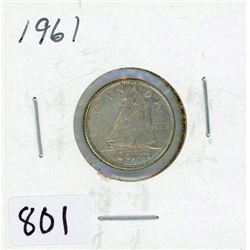801 - TEN CENT COIN (CANADA) *1961* (SILVER)
Schmalz Auctions
TEN CENT COIN (CANADA) *1961* (SILVER)
Bidding Has Concluded

Sold to floor for (2.50) x 1 = 2.50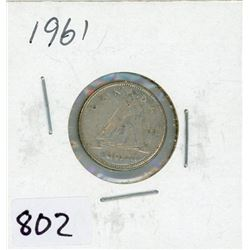802 - TEN CENT COIN (CANADA) *1961* (SILVER)
Schmalz Auctions
TEN CENT COIN (CANADA) *1961* (SILVER)
Bidding Has Concluded

Sold to floor for (2.50) x 1 = 2.50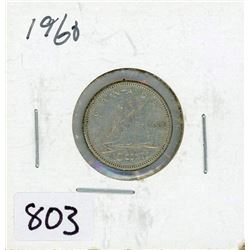803 - TEN CENT COIN (CANADA) *1960* (SILVER)
Schmalz Auctions
TEN CENT COIN (CANADA) *1960* (SILVER)
Bidding Has Concluded

Sold to floor for (2.50) x 1 = 2.50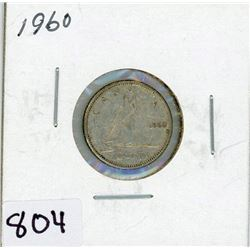804 - TEN CENT COIN (CANADA) *1960* (SILVER)
Schmalz Auctions
TEN CENT COIN (CANADA) *1960* (SILVER)
Bidding Has Concluded

Sold to floor for (2.50) x 1 = 2.50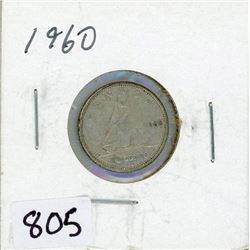805 - TEN CENT COIN (CANADA) *1960* (SILVER)
Schmalz Auctions
TEN CENT COIN (CANADA) *1960* (SILVER)
Bidding Has Concluded

Sold to floor for (2.50) x 1 = 2.50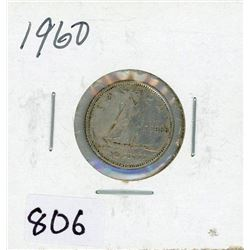806 - TEN CENT COIN (CANADA) *1960* (SILVER)
Schmalz Auctions
TEN CENT COIN (CANADA) *1960* (SILVER)
Bidding Has Concluded

Sold to floor for (2.50) x 1 = 2.50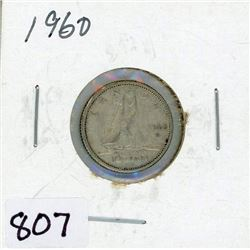807 - TEN CENT COIN (CANADA) *1960* (SILVER)
Schmalz Auctions
TEN CENT COIN (CANADA) *1960* (SILVER)
Bidding Has Concluded

Sold to floor for (2.50) x 1 = 2.50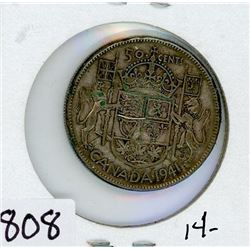808 - FIFTY CENT COIN (CANADA) *1941* (SILVER)
Schmalz Auctions
FIFTY CENT COIN (CANADA) *1941* (SILVER)
Bidding Has Concluded

Sold to tspoon64 for (5.00 + 0.65) x 1 = 5.65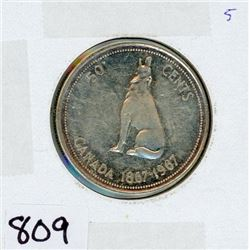809 - FIFTY CENT COIN (CANADA) *1967* (SILVER)
Schmalz Auctions
FIFTY CENT COIN (CANADA) *1967* (SILVER)
Bidding Has Concluded

Sold to tspoon64 for (5.00 + 0.65) x 1 = 5.65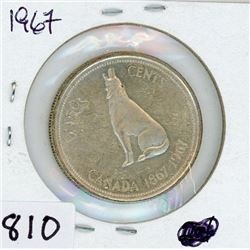810 - FIFTY CENT COIN (CANADA) *1967* (SILVER)
Schmalz Auctions
FIFTY CENT COIN (CANADA) *1967* (SILVER)
Bidding Has Concluded

Sold to tspoon64 for (5.00 + 0.65) x 1 = 5.65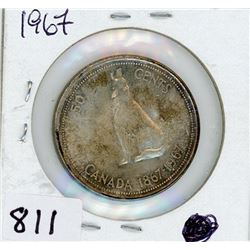811 - FIFTY CENT COIN (CANADA) *1967* (SILVER)
Schmalz Auctions
FIFTY CENT COIN (CANADA) *1967* (SILVER)
Bidding Has Concluded

Sold to tspoon64 for (5.00 + 0.65) x 1 = 5.65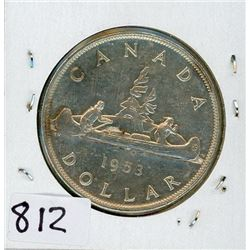812 - ONE DOLLAR COIN (CANADA) *1953* (SILVER)
Schmalz Auctions
ONE DOLLAR COIN (CANADA) *1953* (SILVER)
Bidding Has Concluded

Sold to floor for (45.00) x 1 = 45.00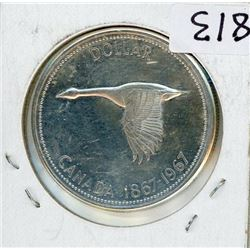813 - ONE DOLLAR COIN (CANADA) *1967* (SILVER)
Schmalz Auctions
ONE DOLLAR COIN (CANADA) *1967* (SILVER)
Bidding Has Concluded

Sold to wildbull for (12.50 + 1.63) x 1 = 14.13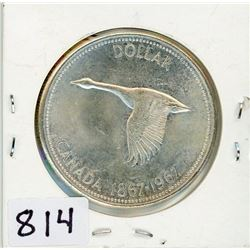814 - ONE DOLLAR COIN (CANADA) *1967* (SILVER)
Schmalz Auctions
ONE DOLLAR COIN (CANADA) *1967* (SILVER)
Bidding Has Concluded

Sold to pickerjed for (15.00 + 1.95) x 1 = 16.95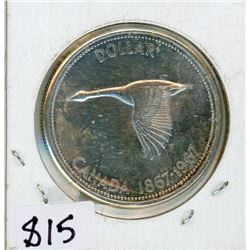815 - ONE DOLLAR COIN (CANADA) *1967* (SILVER)
Schmalz Auctions
ONE DOLLAR COIN (CANADA) *1967* (SILVER)
Bidding Has Concluded

Sold to Fitrnmom2 for (15.00 + 1.95) x 1 = 16.95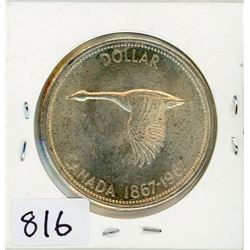816 - ONE DOLLAR COIN (CANADA) *1967* (SILVER)
Schmalz Auctions
ONE DOLLAR COIN (CANADA) *1967* (SILVER)
Bidding Has Concluded

Sold to wildbull for (12.50 + 1.63) x 1 = 14.13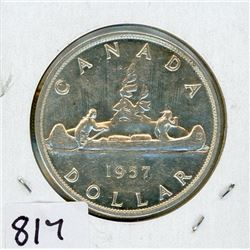817 - ONE DOLLAR COIN (CANADA) *1957* (SILVER)
Schmalz Auctions
ONE DOLLAR COIN (CANADA) *1957* (SILVER)
Bidding Has Concluded

Sold to gameworn for (15.00 + 1.95) x 1 = 16.95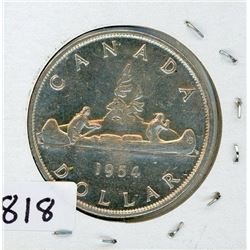818 - ONE DOLLAR COIN (CANADA) *1954* (SILVER)
Schmalz Auctions
ONE DOLLAR COIN (CANADA) *1954* (SILVER)
Bidding Has Concluded

Sold to floor for (47.50) x 1 = 47.50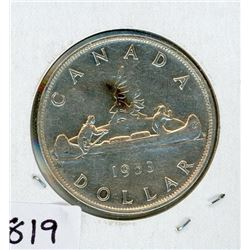819 - ONE DOLLAR COIN (CANADA) *1953* (SILVER)
Schmalz Auctions
ONE DOLLAR COIN (CANADA) *1953* (SILVER)
Bidding Has Concluded

Sold to Fitrnmom2 for (22.50 + 2.93) x 1 = 25.43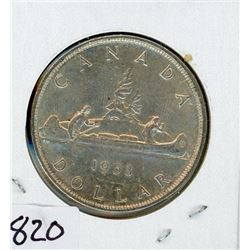820 - ONE DOLLAR COIN (CANADA) *1953* (SILVER)
Schmalz Auctions
ONE DOLLAR COIN (CANADA) *1953* (SILVER)
Bidding Has Concluded

Sold to gameworn for (12.50 + 1.63) x 1 = 14.13821 - ONE DOLLAR COIN (CANADA) *1953* (SILVER)
Schmalz Auctions
ONE DOLLAR COIN (CANADA) *1953* (SILVER)
Bidding Has Concluded

Sold to Fitrnmom2 for (17.50 + 2.28) x 1 = 19.78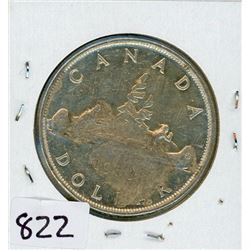822 - ONE DOLLAR COIN (CANADA) *1953* (SILVER)
Schmalz Auctions
ONE DOLLAR COIN (CANADA) *1953* (SILVER)
Bidding Has Concluded

Sold to tspoon64 for (10.00 + 1.30) x 1 = 11.30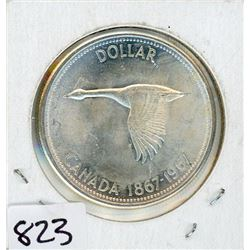823 - ONE DOLLAR COIN (CANADA) *1967* (SILVER)
Schmalz Auctions
ONE DOLLAR COIN (CANADA) *1967* (SILVER)
Bidding Has Concluded

Sold to wildbull for (12.50 + 1.63) x 1 = 14.13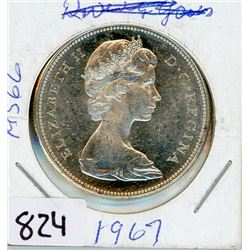824 - ONE DOLLAR COIN (CANADA) *1967* (SILVER)
Schmalz Auctions
ONE DOLLAR COIN (CANADA) *1967* (SILVER)
Bidding Has Concluded

Sold to guardian88 for (15.00 + 1.95) x 1 = 16.95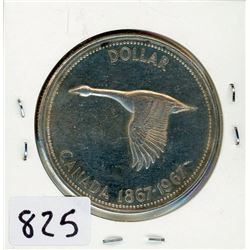825 - ONE DOLLAR COIN (CANADA) *1967* (SILVER)
Schmalz Auctions
ONE DOLLAR COIN (CANADA) *1967* (SILVER)
Bidding Has Concluded

Sold to gameworn for (12.50 + 1.63) x 1 = 14.13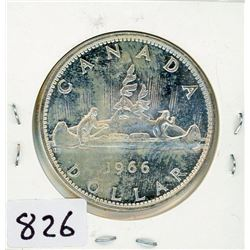826 - ONE DOLLAR COIN (CANADA) *1966* (SILVER)
Schmalz Auctions
ONE DOLLAR COIN (CANADA) *1966* (SILVER)
Bidding Has Concluded

Sold to Lindenguy for (12.50 + 1.63) x 1 = 14.13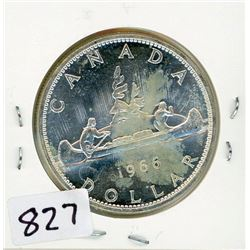827 - ONE DOLLAR COIN (CANADA) *1966* (SILVER)
Schmalz Auctions
ONE DOLLAR COIN (CANADA) *1966* (SILVER)
Bidding Has Concluded

Sold to Lindenguy for (12.50 + 1.63) x 1 = 14.13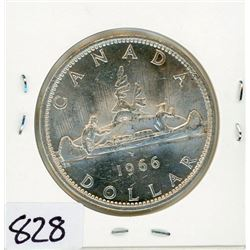828 - ONE DOLLAR COIN (CANADA) *1966* (SILVER)
Schmalz Auctions
ONE DOLLAR COIN (CANADA) *1966* (SILVER)
Bidding Has Concluded

Sold to tspoon64 for (10.00 + 1.30) x 1 = 11.30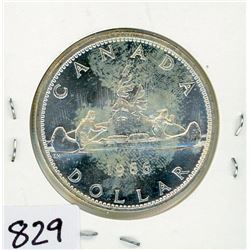829 - ONE DOLLAR COIN (CANADA) *1966* (SILVER)
Schmalz Auctions
ONE DOLLAR COIN (CANADA) *1966* (SILVER)
Bidding Has Concluded

Sold to tspoon64 for (10.00 + 1.30) x 1 = 11.30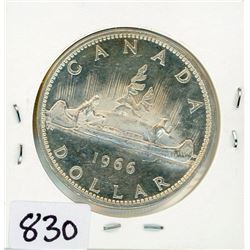830 - ONE DOLLAR COIN (CANADA) *1966* (SILVER)
Schmalz Auctions
ONE DOLLAR COIN (CANADA) *1966* (SILVER)
Bidding Has Concluded

Sold to tspoon64 for (10.00 + 1.30) x 1 = 11.30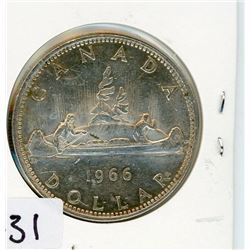831 - ONE DOLLAR COIN (CANADA) *1966* (SILVER)
Schmalz Auctions
ONE DOLLAR COIN (CANADA) *1966* (SILVER)
Bidding Has Concluded

Sold to tspoon64 for (10.00 + 1.30) x 1 = 11.30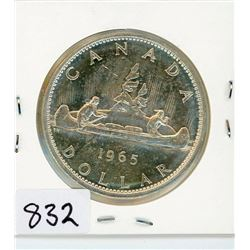832 - ONE DOLLAR COIN (CANADA) *1965* (SILVER)
Schmalz Auctions
ONE DOLLAR COIN (CANADA) *1965* (SILVER)
Bidding Has Concluded

Sold to dottop for (15.00 + 1.95) x 1 = 16.95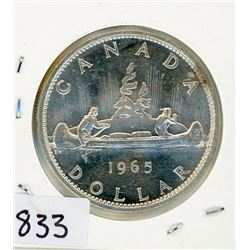833 - ONE DOLLAR COIN (CANADA) *1965* (SILVER)
Schmalz Auctions
ONE DOLLAR COIN (CANADA) *1965* (SILVER)
Bidding Has Concluded

Sold to Fitrnmom2 for (12.50 + 1.63) x 1 = 14.13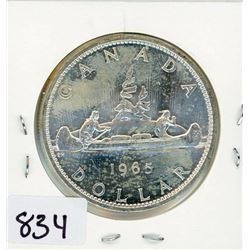834 - ONE DOLLAR COIN (CANADA) *1965* (SILVER)
Schmalz Auctions
ONE DOLLAR COIN (CANADA) *1965* (SILVER)
Bidding Has Concluded

Sold to Lindenguy for (12.50 + 1.63) x 1 = 14.13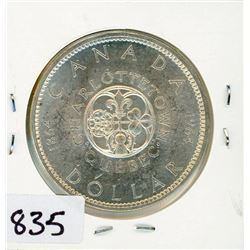835 - ONE DOLLAR COIN (CANADA) *1964* (SILVER)
Schmalz Auctions
ONE DOLLAR COIN (CANADA) *1964* (SILVER)
Bidding Has Concluded

Sold to Argillite for (12.50 + 1.63) x 1 = 14.13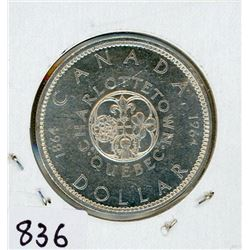836 - ONE DOLLAR COIN (CANADA) *1964* (SILVER)
Schmalz Auctions
ONE DOLLAR COIN (CANADA) *1964* (SILVER)
Bidding Has Concluded

Sold to gameworn for (12.50 + 1.63) x 1 = 14.13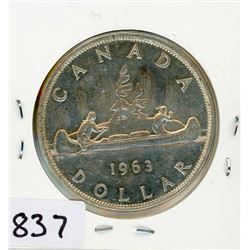837 - ONE DOLLAR COIN (CANADA) *1963* (SILVER)
Schmalz Auctions
ONE DOLLAR COIN (CANADA) *1963* (SILVER)
Bidding Has Concluded

Sold to gameworn for (12.50 + 1.63) x 1 = 14.13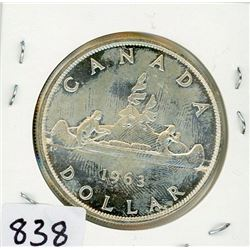838 - ONE DOLLAR COIN (CANADA) *1963* (SILVER)
Schmalz Auctions
ONE DOLLAR COIN (CANADA) *1963* (SILVER)
Bidding Has Concluded

Sold to Argillite for (12.50 + 1.63) x 1 = 14.13839 - ONE DOLLAR COIN (CANADA) *1966* (SILVER)
Schmalz Auctions
ONE DOLLAR COIN (CANADA) *1966* (SILVER)
Bidding Has Concluded

Sold to gameworn for (12.50 + 1.63) x 1 = 14.13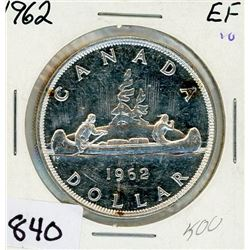840 - ONE DOLLAR COIN (CANADA) *1962* (SILVER)
Schmalz Auctions
ONE DOLLAR COIN (CANADA) *1962* (SILVER)
Bidding Has Concluded

Sold to Fitrnmom2 for (20.00 + 2.60) x 1 = 22.60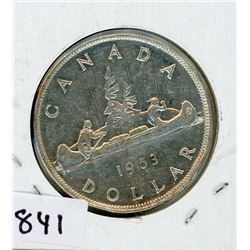841 - ONE DOLLAR COIN (CANADA) *1953* (SILVER)
Schmalz Auctions
ONE DOLLAR COIN (CANADA) *1953* (SILVER)
Bidding Has Concluded

Sold to floor for (30.00) x 1 = 30.00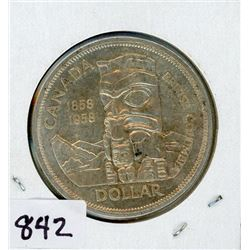842 - ONE DOLLAR COIN (CANADA) *1958* (SILVER)
Schmalz Auctions
ONE DOLLAR COIN (CANADA) *1958* (SILVER)
Bidding Has Concluded

Sold to floor for (17.50) x 1 = 17.50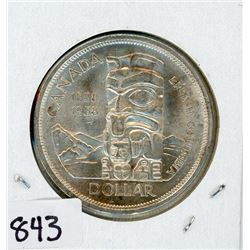843 - ONE DOLLAR COIN (CANADA) *1958* (SILVER)
Schmalz Auctions
ONE DOLLAR COIN (CANADA) *1958* (SILVER)
Bidding Has Concluded

Sold to Lindenguy for (12.50 + 1.63) x 1 = 14.13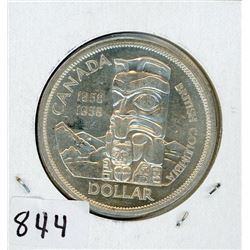844 - ONE DOLLAR COIN (CANADA) *1958* (SILVER)
Schmalz Auctions
ONE DOLLAR COIN (CANADA) *1958* (SILVER)
Bidding Has Concluded

Sold to floor for (22.50) x 1 = 22.50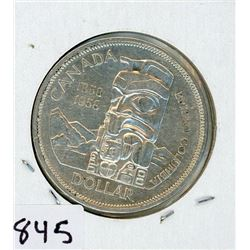845 - ONE DOLLAR COIN (CANADA) *1958* (SILVER)
Schmalz Auctions
ONE DOLLAR COIN (CANADA) *1958* (SILVER)
Bidding Has Concluded

Sold to Lindenguy for (12.50 + 1.63) x 1 = 14.13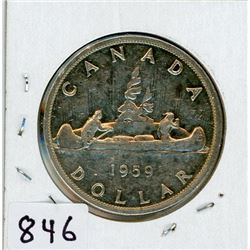846 - ONE DOLLAR COIN (CANADA) *1959* (SILVER)
Schmalz Auctions
ONE DOLLAR COIN (CANADA) *1959* (SILVER)
Bidding Has Concluded

Sold to morris0YF for (17.50 + 2.28) x 1 = 19.78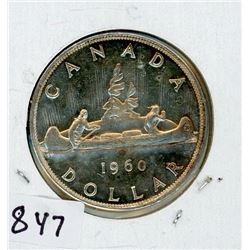847 - ONE DOLLAR COIN (CANADA) *1960* (SILVER)
Schmalz Auctions
ONE DOLLAR COIN (CANADA) *1960* (SILVER)
Bidding Has Concluded

Sold to Argillite for (17.50 + 2.28) x 1 = 19.78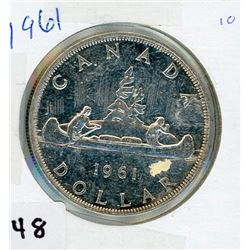848 - ONE DOLLAR COIN (CANADA) *1961* (SILVER)
Schmalz Auctions
ONE DOLLAR COIN (CANADA) *1961* (SILVER)
Bidding Has Concluded

Sold to morris0YF for (17.50 + 2.28) x 1 = 19.78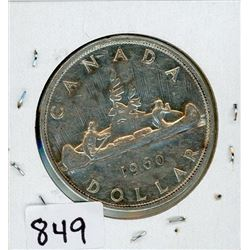849 - ONE DOLLAR COIN (CANADA) *1960* (SILVER)
Schmalz Auctions
ONE DOLLAR COIN (CANADA) *1960* (SILVER)
Bidding Has Concluded

Sold to peterMQA for (15.00 + 1.95) x 1 = 16.95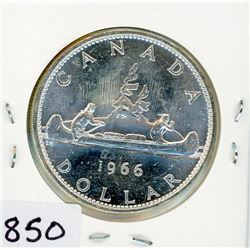850 - ONE DOLLAR COIN (CANADA) *1966* (SILVER)
Schmalz Auctions
ONE DOLLAR COIN (CANADA) *1966* (SILVER)
Bidding Has Concluded

Sold to Gstub for (12.50 + 1.63) x 1 = 14.13